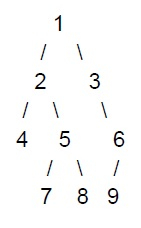# Program to print the nodes at odd levels of a tree using C++

C++Server Side ProgrammingProgramming

#### C in Depth: The Complete C Programming Guide for Beginners

45 Lectures 4.5 hours

#### Practical C++: Learn C++ Basics Step by Step

Most Popular

50 Lectures 4.5 hours

#### Master C and Embedded C Programming- Learn as you go

66 Lectures 5.5 hours

In this tutorial, we will be discussing a program to print the nodes present at the odd levels of a given binary tree.

In this program, the level for the root node is considered as 1 and simultaneously the alternative level is the next odd level.

For example, let us say we are given with the following binary treeThen the nodes at the odd levels of this binary tree will be 1, 4, 5, 6.

## Example

#include <bits/stdc++.h>
using namespace std;
struct Node {
int data;
Node* left, *right;
};
//printing the nodes at odd levels
void print_onodes(Node *root, bool is_odd = true){
if (root == NULL)
return;
if (is_odd)
cout << root->data << " " ;
print_onodes(root->left, !is_odd);
print_onodes(root->right, !is_odd);
}
//creating a new node
struct Node* create_node(int data){
struct Node* node = new Node;
node->data = data;
node->left = node->right = NULL;
return (node);
}
int main(){
struct Node* root = create_node(13);
root->left = create_node(21);
root->right = create_node(43);
root->left->left = create_node(64);
root->left->right = create_node(85);
print_onodes(root);
return 0;
}

## Output

13 64 85